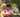### MaxProfit

February 08, 2020

MaxProfit

An array A consisting of N integers is given. It contains daily prices of a stock share for a period of N consecutive days. If a single share was bought on day P and sold on day Q, where 0 ≤ P ≤ Q < N, then the profit of such transaction is equal to A[Q] − A[P], provided that A[Q] ≥ A[P]. Otherwise, the transaction brings loss of A[P] − A[Q].

For example, consider the following array A consisting of six elements such that:
A = 23171
A = 21011
A = 21123
A = 21366
A = 21013
A = 21367
If a share was bought on day 0 and sold on day 2, a loss of 2048 would occur because A − A = 21123 − 23171 = −2048. If a share was bought on day 4 and sold on day 5, a profit of 354 would occur because A − A = 21367 − 21013 = 354. Maximum possible profit was 356. It would occur if a share was bought on day 1 and sold on day 5.

Write a function,
function solution(A);
that, given an array A consisting of N integers containing daily prices of a stock share for a period of N consecutive days, returns the maximum possible profit from one transaction during this period. The function should return 0 if it was impossible to gain any profit.

For example, given array A consisting of six elements such that:
A = 23171
A = 21011
A = 21123
A = 21366
A = 21013
A = 21367
the function should return 356, as explained above.

Write an efficient algorithm for the following assumptions:
• N is an integer within the range [0..400,000];
• each element of array A is an integer within the range [0..200,000].

``````function solution(A) {
// write your code in JavaScript (Node.js 8.9.4)
if(A.length<2) return 0

let min = A
let max = 0
let d
for(let i=0; i<A.length; i++){
if(A[i]<min) min=A[i]
d = A[i]-min
if(d>0 && d>max) max=d
}

return max
}``````Posted by Tai Lu ( 呂台生 ) who's building useful things.You should follow him on Twitter